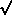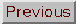### PLANCK LENGTH, PLANCK TIME

Adapted from P. Coles, 1999, The Routledge Critical Dictionary of the New Cosmology, Routledge Inc., New York. Reprinted with the author's permission. To order this book click here: http://www.routledge-ny.com/books.cfm?isbn=0415923549

The theory of general relativity has to be modified if it is to be applied to situations where the matter density is extremely high, in order to take account of the effects of to quantum physics (see also quantum gravity). In cosmology, this basically means that form when quantum effects manifest themselves on the scale of the horizon.

When do we expect quantum corrections to become significant? Of course, in the absence of a complete theory (or even any theory at all) of quantum gravity, it is not possible to give a precise answer to this question. On the other hand, we can make fairly convincing general arguments that yield estimates of the timescales and energy scales where we expect quantum gravitational effects to be large, and for which therefore we should distrust calculations based only on the classical (non-quantum) theory of general relativity. It turns out that the limit of validity of general relativity in the Friedmann models is fixed by the Planck time, which is of the order of 10-43 seconds after the Big Bang.

The Planck time tp, is the time for which quantum fluctuations governed by the Heisenberg uncertainty principle exist on the scale of the Planck length, lp = ctp, where c is the speed of light. From these two scales we can construct other Planck quantities such as the Planck mass, mp, the Planck energy, Ep, and so on. Starting from the Heisenberg uncertainty principle in the formEth / 2and ignoring any factors of 2from now on, we can see that on dimensional grounds alone we can identify the energy term with some mass mp through the relation E = mpc2. Assuming thatt can be represented as the Planck time tp, we have

mpc2tph

We can express the Planck mass as a Planck densityp times a Planck volume (or rather the cube of a Planek length). We want to bring gravity into these considerations, in the shape of the Newtonian gravitational constant G. We can do this by noting that the free-fall collapse time for a self-gravitating body of densityp is given by t2 = 1 / Gp. Replacing m byp(ctp)3 and thenp by 1 / Gtp2 in the above expression leads to

c5tp2 / Gh

which finally leads us to an expression for the Planck time in terms of fundamental constants only:

tp(hG / c5)

which is around 10-43 seconds. The Planck length is simply this multiplied by the speed of light, c, and is consequently around 10-33 cm. This, for example, is about the size of the cosmological horizon at the Planck time (assuming that the concept of a horizon is meaningful at such an early time). The Planck density is phenomenally high: about 1096 grams per cubic centimetre. Interestingly, however, the Planck mass itself is not an outrageous number: mp =(hc / G) = 10-5 g. We can carry on with this approach to calculate the Planck energy (about 1019 GeV) and the Planck temperature (the Planck energy divided by the Boltzmann constant, which gives about 1032 K).

In order to understand the physical significance of the Planck time and all the quantities derived from it, it is useful to think of it in the following manner, which ultimately coincides with the derivation given above. We can define the Compton time for a particle of mass m to be tC = h / mc2; this represents the time for which it is permissible to violate the conservation of energy by an amount equal to the mass of the particle, as deduced from the uncertainty principle. For example, a pair of virtual particles of mass m can exist for a time of about tC. We can also defined the Compton radius of a body of mass m to be equal to the Compton time times the velocity of light: lC = ctC = h / mc. Obviously both these quantities decrease as m increases. These scales indicate when phenomena which are associated with quantum physics are important for an object of a given mass.

Now, the Schwarzschild radius of a body of mass m is given by lS = 2Gm / c2. This represents, to within an order of magnitude, the radius that a body of mass in must have for its rest-mass energy mc2 to equal to its internal gravitational potential energy UGm2 / lS. General relativity leads us to the conclusion that no particle (not even a photon) can escape from a region of radius lS around a body of mass m; in other words, speaking purely in terms of classical mechanics, the escape velocity from a body of mass m and radius lS is equal to the velocity of light. We can similarly define a Schwarzschild time to be the quantity tS = lS / c = 2Gm/c3; this is simply the time taken by light to travel a proper distance lS. A body of mass m and radius lS has a free-fall collapse of the order of tS. Note that both tS and lS increase as m increases.

We can easily verify that, for a mass equal to the Planck mass, the Compton and Schwarzschild times are equal to each other and to the Planck time.

Likewise, the relevant length scales are all equal. For a mass greater than the Planck mass, that is to say for a macroscopic body, tC < tS and lC < lS, and quantum corrections are expected to be negligible in the description of the gravitational interactions between different parts of the body. Here we can describe the self-gravity of the body using general relativity or even, to a good approximation, Newtonian theory. On the other hand, for bodies of the order of the Planck mass, that is to say for microscopic entities such as elementary particles, tC > tS and lC > lS, and quantum corrections will be important in a description of their self-gravity. In the latter case we must use a theory of quantum gravity in place of general relativity or Newtonian gravity.

At the cosmological level, the Planck time represents the moment before which the characteristic timescale of the expansion is such that the cosmological horizon, given roughly by lP, contains only one particle (with mass equal to the Planck mass) for which lClS. On the same grounds as above, we therefore have to take into account quantum effects on the scale of the cosmological horizon.

It is interesting to note the relationship between the Planck quantities and the properties of black holes. According to theory, a black hole of mass M, because of quantum effects, emits Hawking radiation like a black body. The typical energy of photons emitted by the black hole is kT, where the temperature T is given by

Thc3 / 4k G M

The time needed for such a black hole to evaporate completely (i.e. to lose all its rest-mass energy Mc2 via Hawking radiation) is given by

tG2M3 / hc4

By taking these two equations and inserting M = mp, we arrive at the interesting conclusion that a Planck-mass black hole evaporates on a timescale of the order of the Planck time.

These considerations show that quantum gravitational effects are expected to be important not only at a cosmological level at the Planck time, but also continuously on a microscopic scale for processes operating over distances of about lp and times of about tp. In particular, the components of the metric describing space-time geometry will suffer fluctuations of the order of lp / l on a length scale l and of the order of tp / t on a timescale t. At the Planck time, the fluctuations are 100% on the spatial scale lp of the horizon and on the timescale tp of the expansion. We might imagine the Universe at very early times as behaving like a collection of Planck-mass black holes, continually evaporating and recollapsing in a Planck time. This picture is very different from the idealised, perfect-fluid universe described by the Friedmann models, and it would not be surprising if deductions from these equations, such as the existence of a singularity, were found to be invalid in a full quantum description.

FURTHER READING:

Kolb, E.W. and Turner, M.S., The Early Universe (Addison-Wesley, Redwood City, CA, 1990).